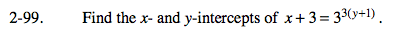### Home > CALC > Chapter 2 > Lesson 2.2.4 > Problem2-99

2-99.

Find the x- and y-intercepts of x + 3 = 33(y+1). Homework Help ✎Find an x-intercept by setting y equal to 0. Find a y-intercept by setting x equal to 0.

y-intercept:
0 + 3 = 33(3y + 1)
3 = 33y + 3

STOP and think! Logarithms could help you solve for y. But there is an easier way...